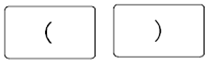## Basic Arithmetic Operations Assignment Help

Assignment Help: >> Calculator Operations - Basic Arithmetic Operations

Basic Arithmetic Operations

This chapter reviews the primary mathematical operations of addition, subtraction, multiplication & division of whole numbers.

APPLY one of the arithmetic operations of addition, subtraction, multiplication & division using whole numbers.

Calculator Usage, Special Keys

This chapter needs the use of the +, -, x, ÷, and = keys.  While using a TI-30 calculator, the number and operation keys are entered as they are written. For instance, the addition of 3 plus 4 is entered as follows:

3 key, + key, 4 key, = key, the answer, 7, is shown

ParenthesesThe parentheses keys permit a complicated equation to be entered as written. This saves the time or effort of rewriting the equation so which multiplication or division is performed first and addition/subtraction is performed second, permitting the problem to be worked from left to right within one pass.

 Adding Whole Numbers Associative law Commutative law Decimal Numbering System Dividing Whole Numbers Hierarchy of Mathematical Operations Multiplying Whole Numbers Subtracting Whole Numbers